# AP Physics B : Using Faraday's Law

## Example Questions

### Example Question #1 : Electricity And Magnetism

An ideal transformer, under a purely resistive load, consists of an iron core that directs all the magnetic flux from a primary winding through a secondary winding. The primary winding has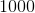closely-packed loops along the coil (such that all see the same magnetic flux). The primary line carries an alternating current at a potential of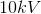. If the secondary line is to have a potential of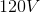, how many closely-packed loops are required in the secondary winding?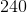Explanation:

In basic consideration, the electrical transformer works directly according to Faraday’s law, following the picture given below.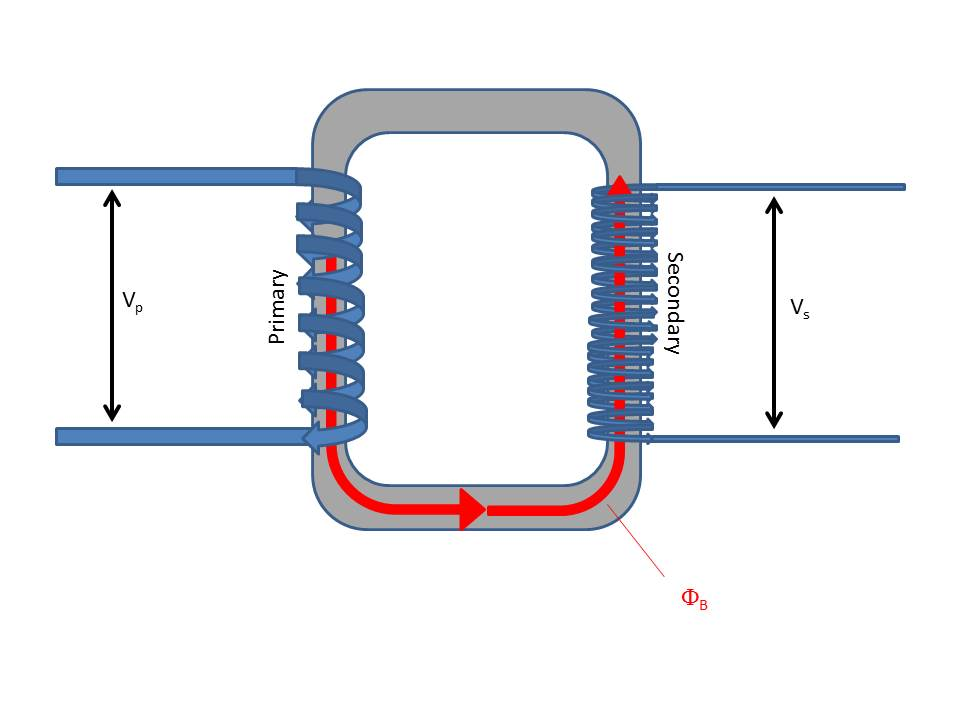If the coils are wound tightly enough that the magnetic flux generated in any of them effectively passes undiminished through all of them, and the iron core directs all the magnetic flux from the primary to secondary coil with insignificant losses, then Faraday’s law points a direct path from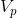to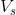. Faraday’s law relates the time of rate of change of magnetic flux to the voltage (emf) around a closed loop: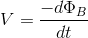If there areloops stacked together, then the total voltage,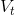, is related to the flux through all loops by: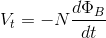From the figure, it is seen that the voltages in each line are dropped over all loops together. Hence, for the primary winding, by Faraday’s law: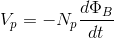For the secondary winding: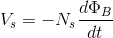Here,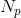and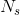are the number of coils in the primary and secondary windings. The quantity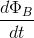is the same in both windings, being transmitted fully (and effectively instantly) by the iron core. This leads to the equation: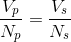This can be used to solve the problem above: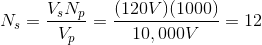NOTE:  This only works under alternating current.  Otherwise, there is no change in current to create the changing magnetic flux on which the transformer depends.

### All AP Physics B Resources